Wed, Oct 04, 2023 @ 03:10 GMT
HomeContributorsTechnical AnalysisTechnical Outlook and Review

# Technical Outlook and Review

USD/JPY:

On the H4, price is bearish biased and moving strongly in an ascending trendline. It is still respecting the Ichimoku indicator, and has already broken the key support level at 131.527. The chart has confirmed a strong downside momentum and is now going to test at 129.898 which coincides with 200% Fibonacci Projection. Alternatively, price could bounce back to test at its previous swing low 131.459 which is also Fibonacci 161.8% Extension

Areas of consideration:

• H4 time frame, 1st resistance at 131.459
• H4 time frame, 1st support at 129.898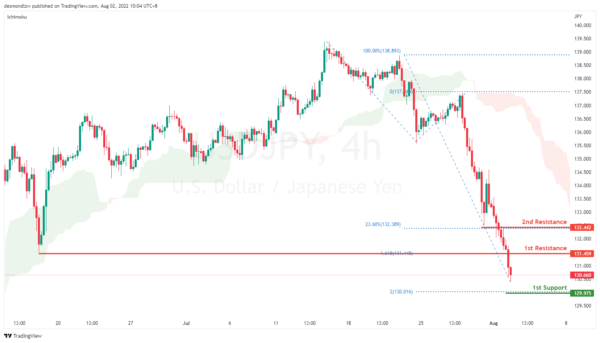DXY:

On the H4, prices have broken the ascending trend into a bearish biased trend. Prices have been strong in its bearish momentum, still respecting the Ichimoku cloud. It is moving towards the 78.6% Fibonacci retracement. If price were to break this support level, it will pull back further to test at 100% Fibonacci level, 103.651 which also coincides with the previous swing lows. Alternatively, price could bounce back and test at the first resistance, 23.6% Fibonacci extension at 106.194

Areas of consideration:

• H4 time frame, 1st resistance at 106.194
• H4 time frame, 1st support at 104.896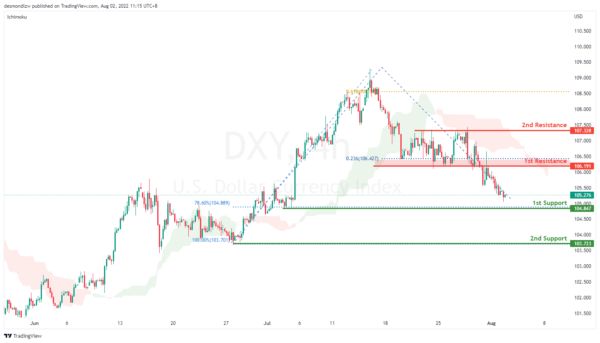EUR/USD :

On the H4, prices have broken the bearish trend moving into a bullish biased trend. Price is now testing at the resistance 1.028, 50% Fibonacci retracement level. If price continues in the ascending trend, it will pullback further to test at the second resistance which is also a key swing low level and 61.8% Fibonacci retracement at 103.6. Alternatively, if prices bounces off the resistance level, it will test at the first support 1.011.

Areas of consideration :

• H4 1st resistance at 1.028
• H4 1st support at 1.014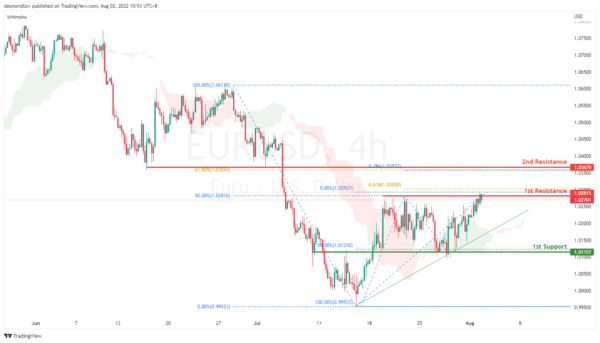GBP/USD:

On the H4, with prices moving in an ascending channel and respecting the Ichimoku cloud, we are bullish biased. Price is now testing the first resistance at 1.232. Once prices break the first resistance and there is upside confirmation, we would expect bullish momentum to carry price to 2nd resistance at 1.240. Alternatively, price could drop to 1st support at 1.217

Areas of consideration:

• H4 1st resistance at 1.232
• H4 1st support at 1.217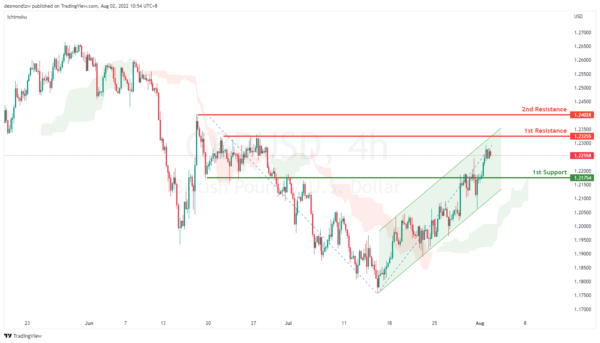USD/CHF:

On the H4, prices are moving in a strong bearish momentum, respecting the descending channel. We have a bearish bias that price might drop to test at our first support, 0.945 which is our key support level. If price breaks reject support, it will pullback to test at 61.8% Fibonacci extension which is still respecting the channel. Price may bounce off from the 1st support to test at the first resistance at 0.953, subsequently testing the second resistance which is at the 23.6% Fibonacci retracement and overlap resistance.

Areas of consideration

• H4 1st resistance at 0.959
• H4 1st support at 0.945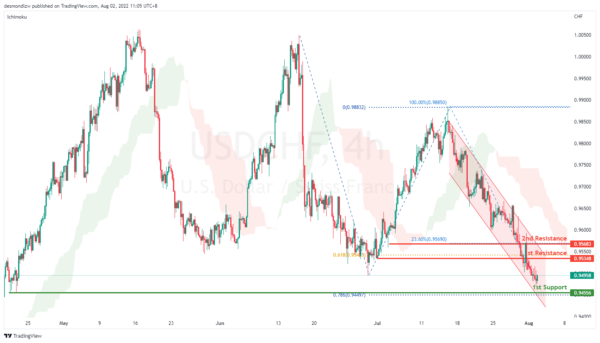XAU/USD (GOLD):

On the H4, with prices going up, we have a bullish bias that price may rise from the 1st resistance at 1785.74 where the the 127.2% projection and 50% fibonacci retracement are to 2nd resistance at 1804.11, which is in line with 61.8% fibonacci retracement. Alternatively, prices could break 1st resistance and drop to 1st support at 1740.70 where the overlap support and 38.2% fibonacci retracement are.

Areas of consideration:

• H4 time frame, 1st Resistance at 1785.74
• H4 time frame, 2nd Resistance at 1820.40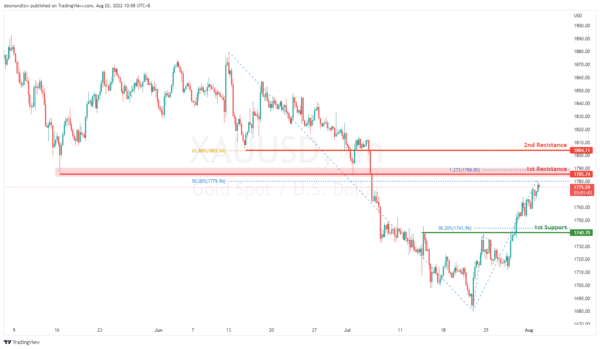AUD/USD:

On the H4, with price moving above the ichimoku cloud and going along the ascending trend channel, we have a bullish bias that price will rise from the 1st resistance at 0.70538, where the 61.8% fibonacci retracement is to the 2nd resistance at 0.71534 at the swing high and 78.6% fibonacci retracement. Alternatively, price may reverse off 1st resistance and drop to the 1st support at 0.68970 at 38.2% fibonacci retracement.

Areas of consideration

• H4 1st resistance at 0.70538
• H4 2nd resistance at 0.71534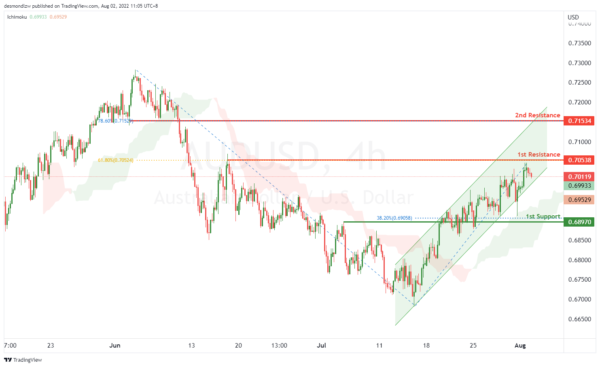NZD/USD:

On the H4, with price going along the ascending trendline, RSI showing an ascending trendline and moving above the ichimoku cloud, we have a bullish bias that price will rise from the 1st resistance at 0.63447 where 61.8% fibonacci projection is to the 2nd resistance at 0.64648 where the 78.6% fibonacci retracement is. Alternatively, price may reverse off the 1st resistance and drop to the 1st support at 0.61924 at the multiple swing lows and 50% fibonacci retracement. Take note the price of 0.62694 could be the intermediate support, as the price is testing this area.

Areas of consideration:

• H4 time frame, 1st resistance at 0.63447
• H4 time frame, 2nd resistance at 0.64648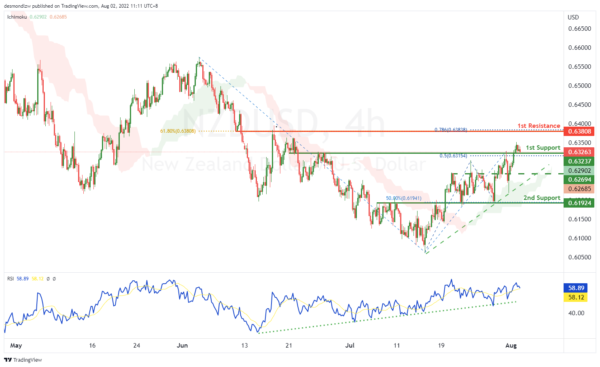On the H4, with the price going along the descending trendline, we have a bearish bias that the price may drop from our 1st resistance at 1.28614, which is in line with swing lows to our 1st support at 1.27652, which is in line with the swing low. Alternatively, the price may rise to the 2nd resistance at 1.29382, which is in line with the overlap resistance and 38.2% fibonacci retracement.

Areas of consideration:

• H4 time frame, 1st resistance at 1.28614
• H4 time frame, 2nd resistance at 1.29382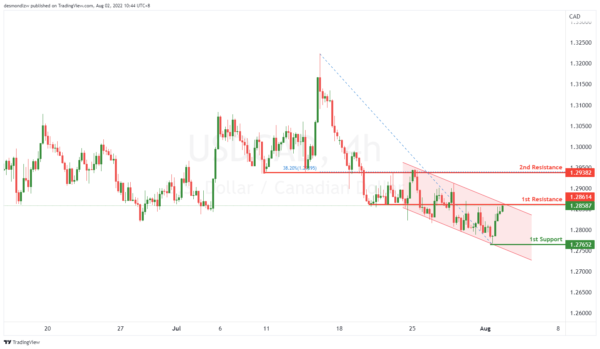OIL:

On the H4, with price moving around the 1st support and 1st resistance, we have a neutral bias that price might drop from the 1st support at 102.799, where the swing low and 23.6% fibonacci retracement are to 2nd support where the swing lows are. Otherwise, the price may rise to our 1st resistance at 108.965, where the overlap resistance is. If the price breaks the 1st resistance, it may rise to the 2nd resistance at 111.464, where the 50% fibonacci retracement is.

Areas of consideration:

• H4 time frame, 1st support of 102.799
• H4 time frame, 2nd support of 99.134

Or

• H4 time frame, 1st resistance of 108.965
• H4 time frame, 1st support of 102.799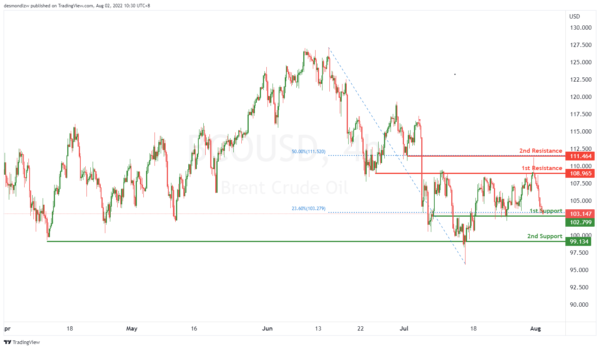Dow Jones Industrial Average:

On the H4, with price moving along an ascending trendline and above the ichimoku indicator, we have a bullish bias that price will rise from our 1st support at 32654 where the pullback support is to the 1st resistance at 33467 where the swing high resistance and -61.8% fibonacci expansion are. Alternatively price could break 1st support structure and drop to 2nd support at 31924 where the pullback support, 38.2% fibonacci retracement and 78.6% fibonacci projection are.

Areas of consideration:

• H4 time frame, 1st resistance of 33467
• H4 time frame, 1st support at 32654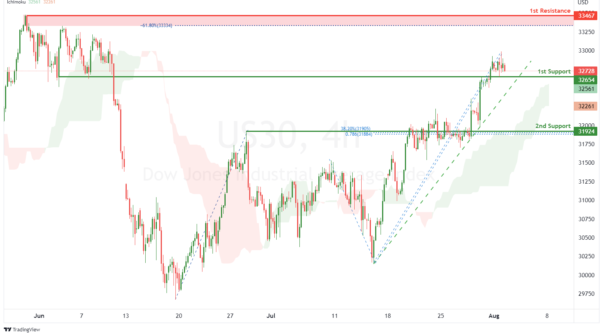DAX:

On the H4, with price moving above the ichimoku indicator, we have a bullish bias that price will rise from 1st support at 13378.95 in line with overlap support to the 1st resistance at 13827.97 where the 61.8% fibonacci retracement and 78.6% fibonacci projection are. Alternatively, price could break 1st support structure and drop to 2nd support at 13026.25 where the pullback support, 50% fibonacci retracement and 100% fibonacci projection are.

Areas of consideration:

• H4 time frame, 1st resistance of 13827.97
• H4 time frame, 1st support at 13378.95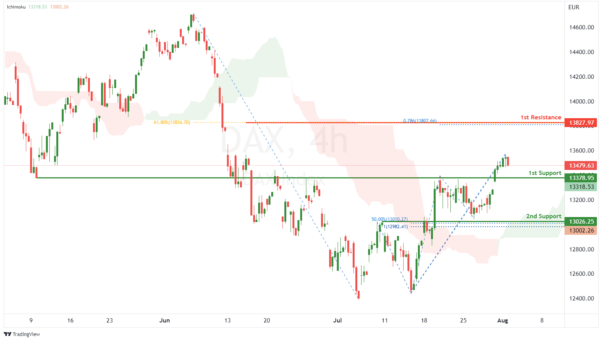ETHUSD:

On the H4, with price moving above the ichimoku indicator, we have a bullish bias that price will rise to our 1st resistance at 1644.27 where the overlap resistance is. Once there is upside confirmation of price breaking 1st resistance structure, we would expect bullish momentum to carry price to 2nd resistance at 1792.30 where the swing high resistance, 127.2% fibonacci extension and 61.8% fibonacci projection are. Alternatively, price could drop to 1st support at 1464.11 where the pullback support, 100% fibonacci projection and 38.2% fibonacci retracement are.

Areas of consideration:

• H4 time frame, 1st resistance of 1644.27
• H4 time frame, 1st support at 1464.11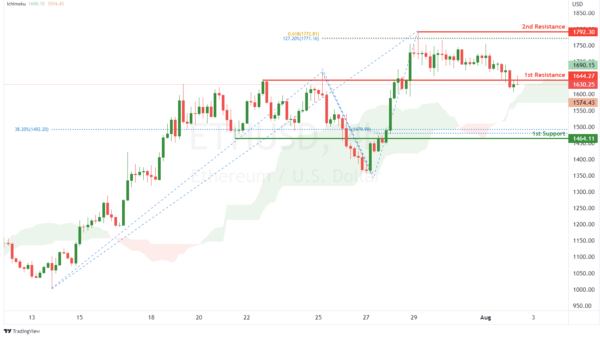BTCUSD:

On the H4, with price moving within a bullish channel and above the ichimoku indicator, we have a bullish bias that price will rise from our 1st support at 22560.82 where the pullback support, 50% fibonacci retracement and 61.8% fibonacci projection are to the 1st resistance at 24331.68 where the pullback resistance is. Alternatively, price could break 1st support structure and drop to 2nd support at 20716.80 where the swing low support and 100% fibonacci projection are.

Areas of consideration:

• H4 time frame, 1st resistance of 24331.68
• H4 time frame, 1st support at 22560.82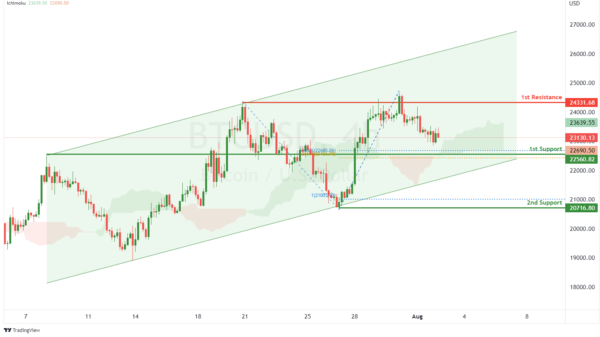S&P 500:

On the H4, with price moving above the ichimoku indicator and within an ascending channel, we have a bullish bias that price will rise from our 1st support at 4087.733 where the pullback support is to the 1st resistance at 4182.677 where the swing high resistance and 100% fibonacci projection are. Alternatively, price could break 1st support and drop to 2nd support at 4014.714 where the pullback support and 23.6% fibonacci retracement are.

Areas of consideration:

• H4 time frame, 1st resistance of 4182.677
• H4 time frame, 1st support at 4087.733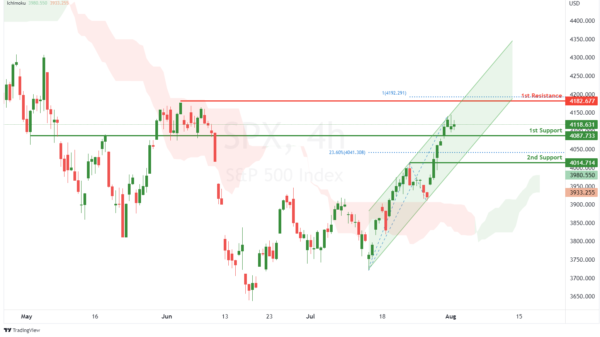IC Marketshttp://www.icmarkets.com/
IC Markets is revolutionizing on-line forex trading; on-line traders are now able to gain access to pricing and liquidity previously only available to investment banks and high net worth individuals.